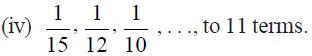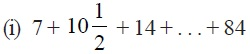Study Materials: ncert solutions

Our ncert solutions for Exercise 5.3 Class 10 maths 5. Arithmetic Progressions - ncert solutions - Toppers Study is the best material for English Medium students cbse board and other state boards students.

Solutions ⇒ Class 10th ⇒ Mathematics ⇒ 5. Arithmetic Progressions

# Exercise 5.3 Class 10 maths 5. Arithmetic Progressions - ncert solutions - Toppers Study

Topper Study classes prepares ncert solutions on practical base problems and comes out with the best result that helps the students and teachers as well as tutors and so many ecademic coaching classes that they need in practical life. Our ncert solutions for Exercise 5.3 Class 10 maths 5. Arithmetic Progressions - ncert solutions - Toppers Study is the best material for English Medium students cbse board and other state boards students.

## Exercise 5.3 Class 10 maths 5. Arithmetic Progressions - ncert solutions - Toppers Study

CBSE board students who preparing for class 10 ncert solutions maths and Mathematics solved exercise chapter 5. Arithmetic Progressions available and this helps in upcoming exams 2023-2024.

### You can Find Mathematics solution Class 10 Chapter 5. Arithmetic Progressions

• All Chapter review quick revision notes for chapter 5. Arithmetic Progressions Class 10
• NCERT Solutions And Textual questions Answers Class 10 Mathematics
• Extra NCERT Book questions Answers Class 10 Mathematics
• Importatnt key points with additional Assignment and questions bank solved.

NCERT Solutions do not only help you to cover your syllabus but also will give to textual support in exams 2023-2024 to complete exercise 5.3 maths class 10 chapter 5 in english medium. So revise and practice these all cbse study materials like class 10 maths chapter 5.3 in english ncert book. Also ensure to repractice all syllabus within time or before board exams for ncert class 10 maths ex 5.3 in english.

See all solutions for class 10 maths chapter 5 exercise 5 in english medium solved questions with answers.

### Exercise 5.3 class 10 Mathematics Chapter 5. Arithmetic Progressions

• Exercise 5.3 Class 10 Maths 5. Arithmetic Progressions - Ncert Solutions - Toppers Study
• Class 10 Ncert Solutions
• Solution Chapter 5. Arithmetic Progressions Class 10
• Solutions Class 10
• Chapter 5. Arithmetic Progressions Exercise 5.3 Class 10

## Exercise 5.3 Class 10 maths 5. Arithmetic Progressions - ncert solutions - Toppers Study

Exercise 5.3

Q1. Find the sum of the following APs:
(i) 2, 7, 12, . . ., to 10 terms.

(ii) –37, –33, –29, . . ., to 12 terms.
(iii) 0.6, 1.7, 2.8, . . ., to 100 terms.Q2. Find the sums given below :(ii) 34 + 32 + 30 + . . . + 10
(iii) –5 + (–8) + (–11) + . . . + (–230)
Q3. In an AP:
(i) given a = 5, d = 3, an = 50, find n and Sn.
(ii) given a = 7, a13 = 35, find d and S13.
(iii) given a12 = 37, d = 3, find a and S12.
(iv) given a3 = 15, S10 = 125, find d and a10.
(v) given d = 5, S9 = 75, find a and a9.
(vi) given a = 2, d = 8, Sn = 90, find n and an.
(vii) given a = 8, an = 62, Sn = 210, find n and d.
(viii) given an = 4, d = 2, Sn = –14, find n and a.
(ix) given a = 3, n = 8, S = 192, find d.
(x) given l = 28, S = 144, and there are total 9 terms. Find a.

Q4. How many terms of the AP : 9, 17, 25, . . . must be taken to give a sum of 636?
Q5. The first term of an AP is 5, the last term is 45 and the sum is 400. Find the number of terms and the common difference.
Q6. The first and the last terms of an AP are 17 and 350 respectively. If the common difference is 9, how many terms are there and what is their sum?
Q7. Find the sum of first 22 terms of an AP in which d = 7 and 22nd term is 149.
Q8. Find the sum of first 51 terms of an AP whose second and third terms are 14 and 18 respectively.
Q9. If the sum of first 7 terms of an AP is 49 and that of 17 terms is 289, find the sum of first n terms.

##### Other Pages of this Chapter: 5. Arithmetic Progressions

Important Study materials for classes 06, 07, 08,09,10, 11 and 12. Like CBSE Notes, Notes for Science, Notes for maths, Notes for Social Science, Notes for Accountancy, Notes for Economics, Notes for political Science, Noes for History, Notes For Bussiness Study, Physical Educations, Sample Papers, Test Papers, Mock Test Papers, Support Materials and Books.Mathematics Class - 11th

NCERT Maths book for CBSE Students.

books

## Study Materials List:

##### Solutions ⇒ Class 10th ⇒ Mathematics
1. Real Numbers
2. Polynomials
3. Pair of Linear Equations in Two Variables
5. Arithmetic Progressions
6. Triangles
7. Coordinate Geometry
8. Introduction to Trigonometry
9. Some Applications of Trigonometry
10. Circles
11. Constructions
12. Areas Related to Circles
13. Surface Areas and Volumes
14. Statistics
15. Probability

New Books